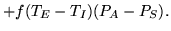Next: 5.3 Comparison of Basic Up: 5 Evaluation Results Previous: 5.1 Experimental Workloads

## 5.2 Evaluation Methodology

Table 6:  Logged data and static parameters for calculating energy consumption.Total elapsed time in the systemTotal elapsed idle time in the systemTotal time that node j operates in Standby mode while process i is activeTotal time that node j operates in Nap mode while process i is activeActivity factor of memory transactions3Set of all processes in the systemPower dissipation of a node in Read/Write modePower dissipation of a node in Standby modePower dissipation of a node in Nap modeSet of all nodes in the system

Our PAVM implementation is currently fully operational, and has all the means to communicate with the RDRAM memory controller (i82850 chipset) on our Pentium 4 testbed to manage power by controlling the power state of individual nodes. However, due to a hardware bug found in the chipset , the system will hang when instructed to put a node in Nap mode. As a result, this prevents us from directly measuring the actual energy saved, e.g., with a digital power meter. However, by logging detailed information about the state of processes and the state of the system, combined with the information from memory device's datasheet, we can calculate fairly accurately how much energy would be consumed.

Specifically, to accurately calculate energy consumption, we need to log the operating times and memory use characteristics shown in the top portion of Table 6 from the running system. We also need some static system/memory parameters, shown in the bottom portion of Table 6, to complete the energy calculation.

Using these parameters, we can compute the energy consumed with the following equation:(1)

whereor, depending on the power-management scheme used (see next section). This equation consists of three terms. The first is the energy consumed by the memory while the system is idle, and is simply the product of the number of nodes, total idle time, and eitheror, depending on whether the nodes are kept in Nap or Standby modes when system is idle. The second term computes the energy for keeping nodes in Nap and Standby modes while the system is not idle. This is a double summation over all processes and all nodes, where we weight the total time a particular process keeps a particular node in Nap and Standby modes byand, respectively. The last term reflects the additional energy required to actually read/write data from/to a memory device in Standby mode, and is a product of the total non-idle time, the additional power dissipated in Read/Write mode over Standby, and an activity factor,, that gives the total number of memory transactions as a fraction of the maximum number possible when a device is kept in Read/Write mode (i.e., peak memory bandwidth).Next: 5.3 Comparison of Basic Up: 5 Evaluation Results Previous: 5.1 Experimental Workloads
2003-03-03